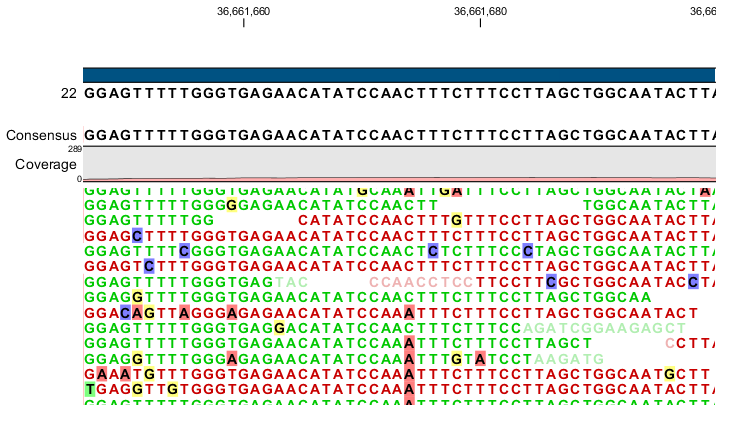# Probabilistic variant detection

The purpose of the Probabilistic Variant Caller is the calling of variants from a read mapping using a probabilistic model. In comparison to other available variant callers, it can detect variants in data sets from haploid (e.g. Bacteria), diploid (e.g. Human) and polyploid organisms (e.g. Cancer and higher plants) with a high sensitivity and specificity.

The algorithm is a combination of a Bayesian model and a Maximum Likelihood approach to calculate prior and error probabilities for the Bayesian model. Parameters are calculated on the mapped reads alone without considering the reference sequence. After these values have been calculated, the probability for each combination of alleles (e.g. A/G) after observing a certain combination of nucleotides from the reads at every position in the genome will be determined. This probability is then used to find out which of the allele combinations (e.g. A/G) is the most likely one for each position. This can then be compared with the reference allele to find out if it is different from the reference sequence and therefore can be called as a variant.

Note: In the current version, the probabilistic variant detection is not designed to detect minor variants (like rare alleles) with a frequency of less than 15%. If you are expecting a allele frequency of less than 15% we recommend to use a higher ploidy level as parameter or apply the quality-based variant detection algorithm (see Quality-based variant detection) with a post-filtering step for average base quality and forward-reverse read balance.Figure 26.12: An example of a heterozygous variant surrounded by a lot of noise from sequencing errors.

Subsections